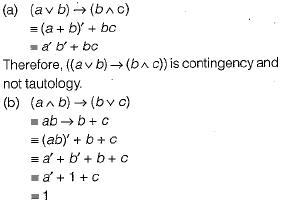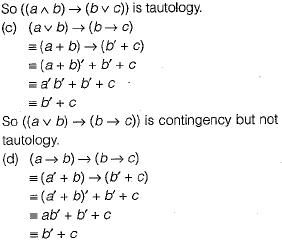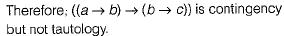Courses

# Mathematical Logic (Basic Level) - 1

## 10 Questions MCQ Test Question Bank for GATE Computer Science Engineering | Mathematical Logic (Basic Level) - 1

Description
This mock test of Mathematical Logic (Basic Level) - 1 for GATE helps you for every GATE entrance exam. This contains 10 Multiple Choice Questions for GATE Mathematical Logic (Basic Level) - 1 (mcq) to study with solutions a complete question bank. The solved questions answers in this Mathematical Logic (Basic Level) - 1 quiz give you a good mix of easy questions and tough questions. GATE students definitely take this Mathematical Logic (Basic Level) - 1 exercise for a better result in the exam. You can find other Mathematical Logic (Basic Level) - 1 extra questions, long questions & short questions for GATE on EduRev as well by searching above.
QUESTION: 1

### Which of the following is/are tautology:​

Solution:

(a) (a v b)→(b ∧ c)
= (a + b)'+ bc
= a' b' + bc
Therefore, ((a v b) → (b ∧ c)) is contingency and not tautology.
(b) (a ∧ b) →(b v c)
= ab → b + c
= (ab)' + b + c
= a' + b' + b + c
= a' + 1 + c
= 1
So ((a ∧ b) →(b v c)) is tautology,
(c) (a v b)→ (b → c)
= (a + b) → (b' + c)
= (a+ b)' + b' + c
= a' b' + b' + c
= b' + c
So ((a v b) → (b → c)) is contingency but not tautology.
(d) (a → b) → (b→ c)
= (a' + b) → (b' + c)
= (a' + b) + b' + c
= ab' + b' + c
= b' + c
Therefore; ((a → b) → (b → c)} is contingency but not tautology.

QUESTION: 2

### ​If the proposition¬ p ⇒ q is true, then the truth value of the proposition ¬ p v (p ⇒ q), where ¬ is negation, 'v' is inclusive or and '⇒’ is implication, is

Solution: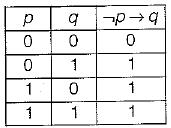Now since ¬ p → q is given true, we reduce the truth table as follows: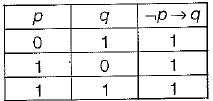In the reduced truth table we need to find the truth value of ¬ pv(p→q} = p' +(p → q)
≡ p' + p' + q ≡ p' + q
The truth value of p' + q in the reduced truth table is given below: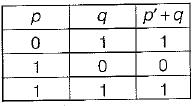Since in the reduced truth table also, the given expression is sometimes true and sometimes false, therefore the truth value of proposition ¬p v (p → q) can not be determined.

QUESTION: 3

### Let a, b, c, d be propositions. Assume that the equivalence a ⇔ ( bv ¬ b) and b ⇔ c hold. Then the truth-value of the formula (a ∧ b) → (a ∧ c) v d is always

Solution:

a ⇔ (b v ¬ b )
a ⇔ 4 True
So a is true, i.e. a = 1
b ⇔ c holds. So b = c
Now the given expression is
(a ∧ b) → ((a ∧ c) v d) ≡ (a - b ) → ((a . c) + d)
Putting a = 1 in above expression we get
1 • b → ((1 • c) + d)
≡ b→ c + d
≡ b' + c+ d
Now putting b = c in above expression we get
≡ c' + c+ d=1 + d=1
So the expression is always true.

QUESTION: 4

The following propositional statement is
(P → (Q v R)) → (( P ∧ Q) → R)

Solution:

(P → (Q v R)) → ((P ∧ Q) → R)
≡ (P→ Q+ R) → (PQ → R)
≡ [P' + Q + R] → [(PQ)' + R]
≡ [P' + Q + R] → [P' + Q' + R]
≡ (P' + Q + R)' + P' + Q' + R
≡ PQ' R' + P' + Q' + R
≡ Q' + Q' PR' + P' + R
≡ Q' + P' + R (by absorption law)
Which is a contingency (i.e. satisfiable but not valid).

QUESTION: 5

A logical binary relation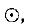is defined as follows: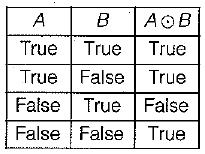Let ~ be the unary negation (NOT) operator, with higher precedence, thanWhich one of the following is equivalent to A ∧ B?

Solution:

By using min terms we can define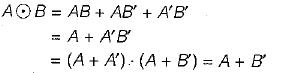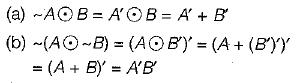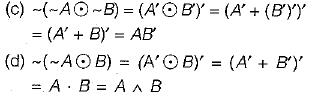∴ Only, choice (d) = A ∧ B
[Note: This problem can also be done by constructing truth table for each choice and - comparing with truth table for A ∧ B]

QUESTION: 6

Which of the following is TRUE about formulae in Conjunctive Normal Form?

Solution:

In conjunction normal form, for any particular assignment of truth values, all except one clause will always evaluate to true. So, the proportion of clauses which evaluate to true to the total number of clauses is equal to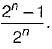Now putting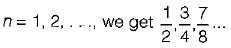All of these proportions are ≥ 1/2 and so choice (a) atleast half of the clauses evaluate to true, is. the correct answer.

QUESTION: 7

The binary operation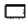is defined as follows: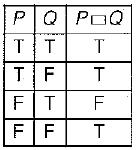Which one of the following is equivalent to P v Q?

Solution:

The given table can be converted into boolean function by adding mrnterms corresponding to true rows.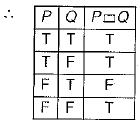Since there is only one false in the above truth table, we can represent the function PQ more efficiently, in conjunctive normal form.
Translates PQ = P + Q' (the max -term corresponding to the third row, where the function is false).
Now, we can easily translate the choices into boolean algebra as follows: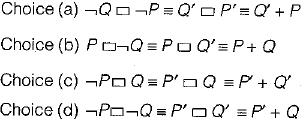As we can clearly see only choice (b)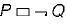is equivalent to P+ Q.

QUESTION: 8

Consider the following well-formed formulae: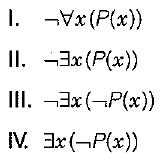Which of the above are equivalent?

Solution: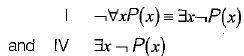Clearly, choices I and IV are equivalent.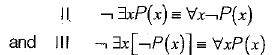Clearly II and III are not equivalent to each other or to I and IV.

QUESTION: 9

Which one of the following is NOT logically e quivalent to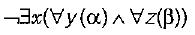Solution:
QUESTION: 10

The CORRECT formula for the sentence, "not all rainy days are cold” is

Solution: